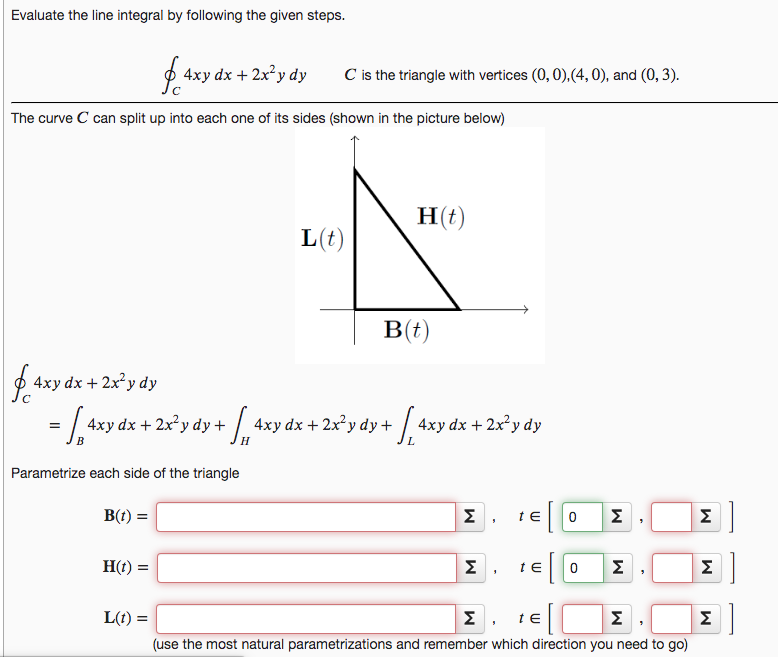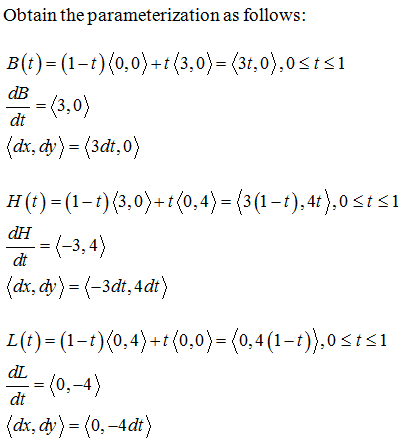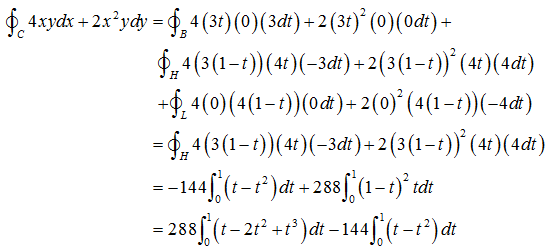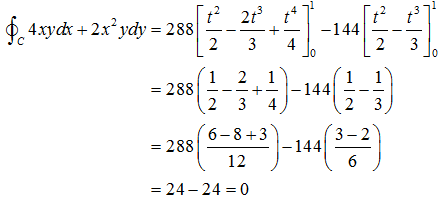# Evaluate the line integral by following the given steps.4xy dx + 2x²y dyC is the triangle with vertices (0,0),(4, 0), and (0, 3).The curve C can split up into each one of its sides (shown in the picture below)Н)L(t)B(t)4xy dx + 2x²y dy4хydx + 2x*y dy +4xy dx + 2x² y dy+ | 4xy dx + 2x²y dyНParametrize each side of the triangleB(t) =ΣΣΣН()ΣΣΣL(t) =Σ . teΣΣ(use the most natural parametrizations and remember which direction you need to go)

Question
19 viewshelp_outlineImage TranscriptioncloseEvaluate the line integral by following the given steps. 4xy dx + 2x²y dy C is the triangle with vertices (0,0),(4, 0), and (0, 3). The curve C can split up into each one of its sides (shown in the picture below) Н) L(t) B(t) 4xy dx + 2x²y dy 4хydx + 2x*y dy + 4xy dx + 2x² y dy+ | 4xy dx + 2x²y dy Н Parametrize each side of the triangle B(t) = Σ Σ Σ Н() Σ Σ Σ L(t) = Σ . te Σ Σ (use the most natural parametrizations and remember which direction you need to go) fullscreen
check_circle

Solution:The line integral becomesOn further computation,...

### Want to see the full answer?

See Solution

#### Want to see this answer and more?

Solutions are written by subject experts who are available 24/7. Questions are typically answered within 1 hour.*

See Solution
*Response times may vary by subject and question.
Tagged in